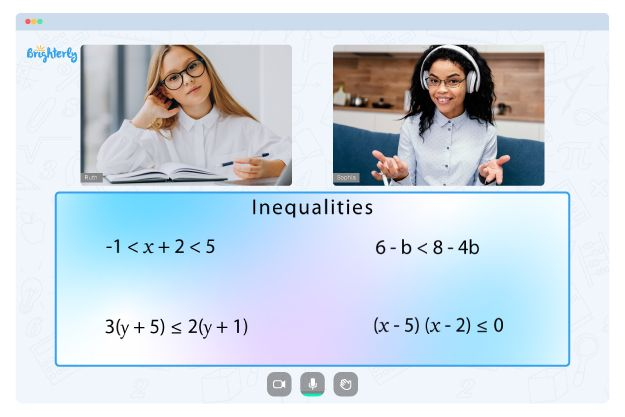# Graphing Inequalities on a Number Line Worksheet

Inequalities on a number line are mathematical statements that compare the relative size of two numbers using symbols such as “greater than” (>), “less than” (<), “greater than or equal to” (>=), and “less than or equal to” (<=). These symbols can represent a range of numbers on a number line rather than a single value. The inequalities on a number line worksheet guides students through solving one-variable inequalities and then graphing those results. Teachers recommend it for students looking to practice various arithmetic concepts.

## Free graphing inequalities number line worksheet

Using the inequality number line worksheet can help students understand the real world. Those who dream of careers in surveying, game design, and even education will benefit from using this tool to learn in their early years. The worksheet is free on Brighterly and regularly updated to include the latest and most relevant examples in line with standard educational practices.

Math for Kids

Is Your Child Struggling With Math?
1:1 Online Math TutoringKids need to understand how inequities play out in the actual world, and they may do so via comparisons. For instance, “James is shorter than John” or “My school is bigger than yours.” The questions in the inequalities number line worksheet serve as the numerical equivalent of such real-life comparisons.

You can get the printable version of the graph inequalities on a number line worksheet to aid independent continuous learning. As kids continue to learn with the sheet, they will develop a mastery of the concept.### Graphing Inequalities on a Number Line Worksheet PDF

Graph Inequalities On A Number Line Worksheet### Graphing Inequalities on a Number Line Worksheet PDF

Graphing Inequalities Number Line Worksheet### Graphing Inequalities on a Number Line Worksheet PDF

Graphing Inequalities On A Number Line Worksheet### Graphing Inequalities on a Number Line Worksheet PDF

Inequalities On A Number Line Worksheet

Problems with Inequalities?• Does your child need extra help with understanding inequalities concepts?
• Start studying with an online tutor.

Is your child finding it hard to grasp of inequalities? An online tutor could be of assistance.